# Sample Data: Shapley Supercluster Galaxies

Locations of Shapley supercluster galaxies annotated with magnitude, velocity (in km/s), and error marks

## Details

Locations of Shapley supercluster galaxies in a polygonal observation region bounded by the Rectangle[{192.802, -37.7551}, {216.262, -27.4066}] degrees, annotated with magnitude, velocity (in km/s), and error marks.

## Examples

### Basic Examples (2)

Retrieve the data:

 In:=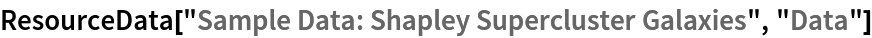Out=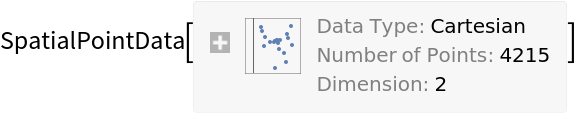Summary of the spatial point data:

 In:=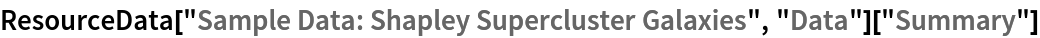Out=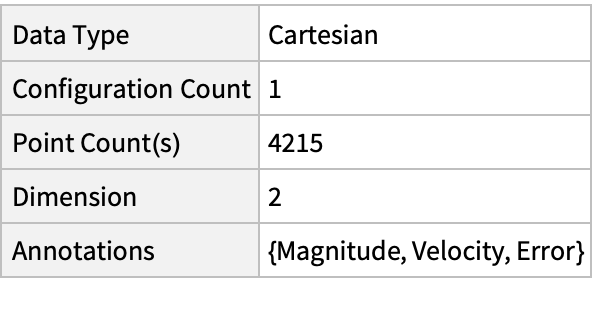### Visualizations (3)

Plot the spatial point data:

 In:=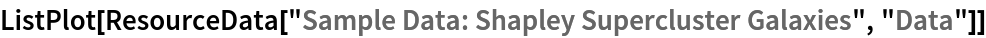Out=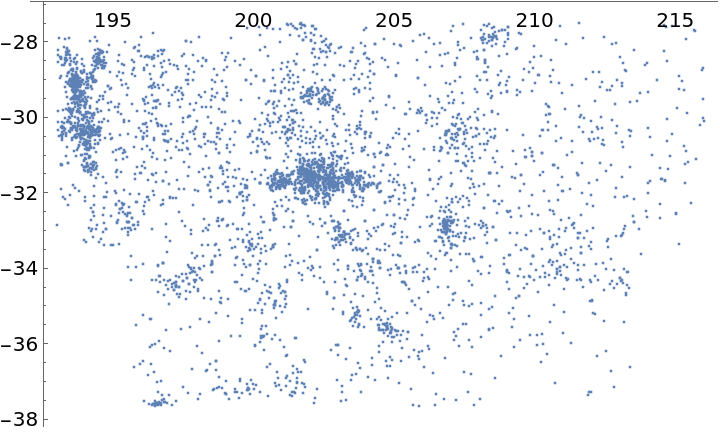Visualize the points with magnitude annotations:

 In:=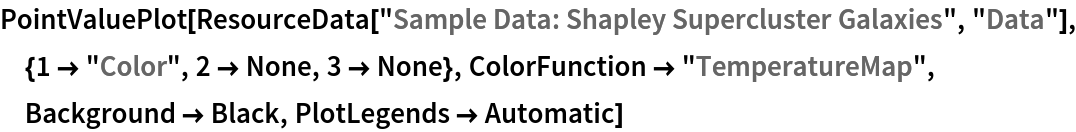Out=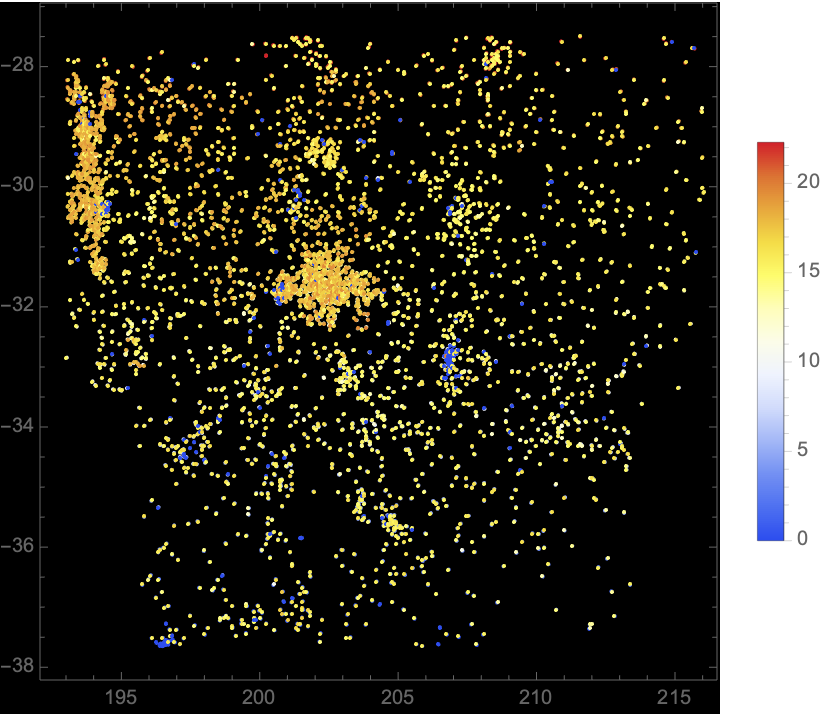Visualize the smooth point density of the data:

 In:=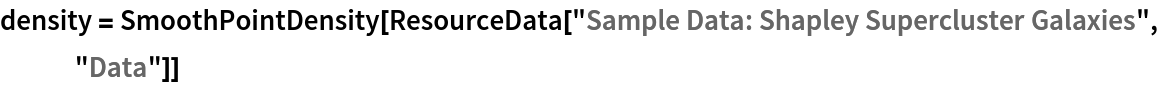Out=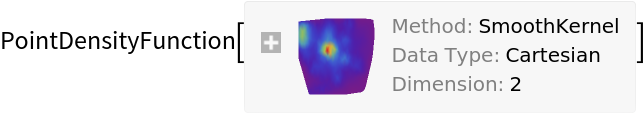In:=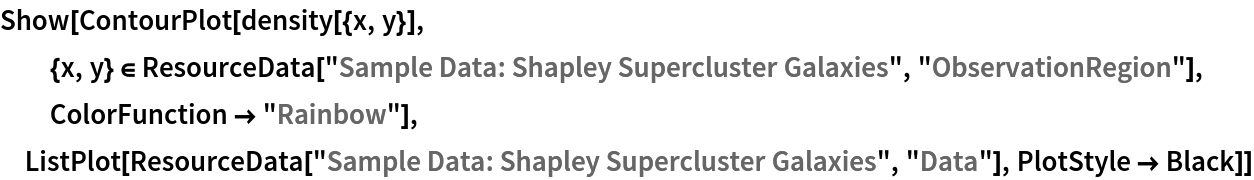Out=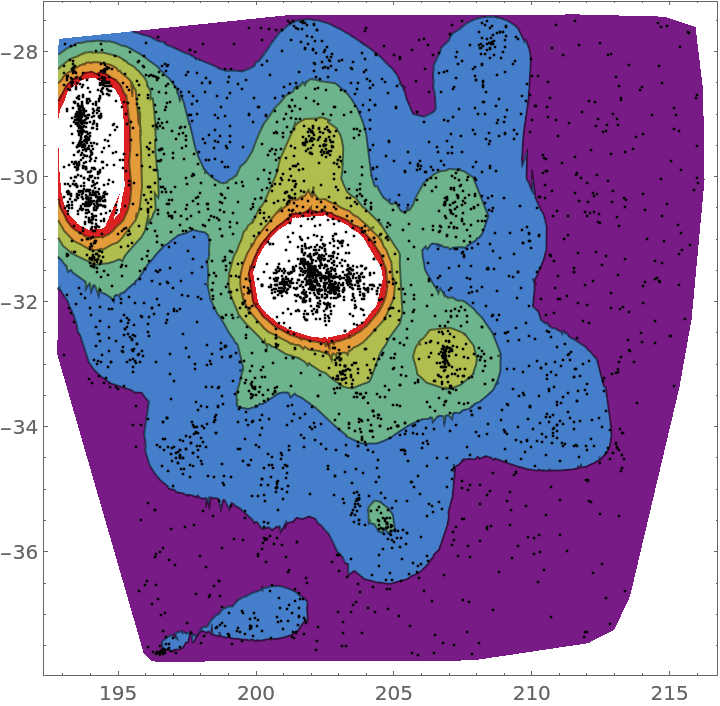### Analysis (3)

Compute probability of finding a point within given radius of an existing point - NearestNeighborG is the CDF of the nearest neighbor distribution:

 In:=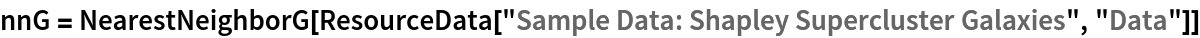Out=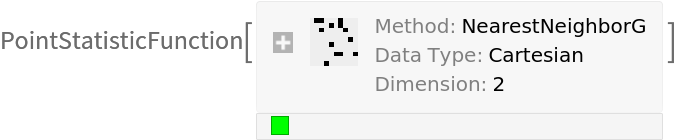In:=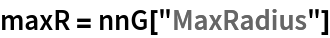Out=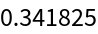In:=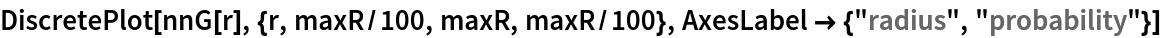Out=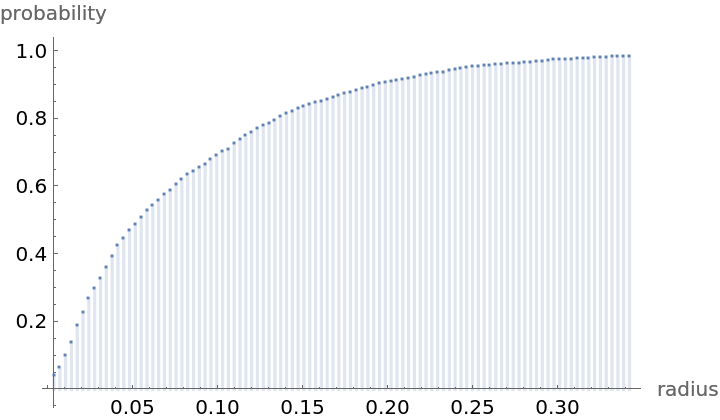Mean distance between a typical point and its nearest neighbor (for positive support distribution can be approximated via a Riemann sum of 1-CDF):

 In:=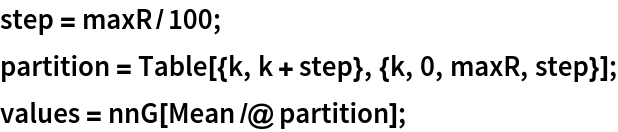In:=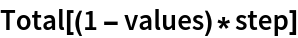Out=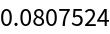Test for complete spacial randomness:

 In:=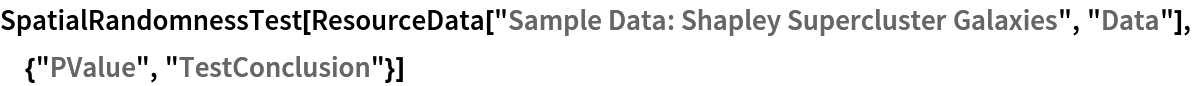Out=Gosia Konwerska, "Sample Data: Shapley Supercluster Galaxies" from the Wolfram Data Repository (2021)